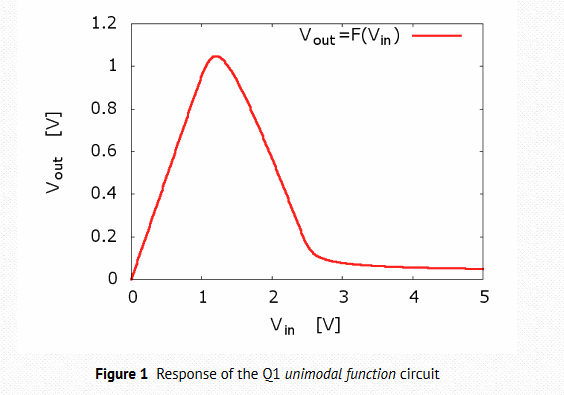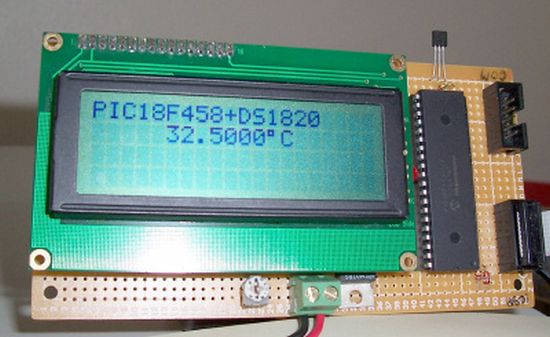Iterated-map circuit creates chaos

the Design Idea circuit shown below is a simple implementation of an iterated unimodal map, reminiscent of the logistic or Verhulst map encountered in the study of nonlinear dynamics. It is useful to show chaotic discrete-time dynamics to students, or as a random number generator. Specifically, the circuit implements: Vk+1 = rF(Vk), where F is a nonlinear unimodal function (a “bump”), implemented by the circuit in the dashed box. The response of this circuit is shown in the Vout vs. Vin plot.

r is a gain factor provided by U1b. U1a and U3a are simply buffers. During the low phase of the clock signal, the output voltage of the nonlinear function is stored on C1. When the clock switches to high, this voltage is transferred to C2, and then used as the next input to the unimodal function. The supply voltage is 12V. S3 (¼ 4066) is used as an inverter.For low gain settings (r), the iterates have the origin, zero volts, as stable equilibrium: V* = rF(V*). By increasing the gain, the circuit moves from a stable equilibrium to n-periodic oscillations. The iterates of an n-periodic oscillation can be seen as equilibria of the n-iterated map, e.g., V* = rF(rF(rF(V*))) for 3-periodic oscillations. This is shown in the first oscilloscope screenshot, for r=4.7

For More Details : Iterated-map circuit creates chaos

Current Project / Post can also be found using:

• pic microcontroller chaotic map